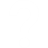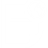# Quiz## Review questions

Assess your understanding of this learning pathway by selecting the correct answer for each of the following questions:

• Scientific hypotheses are ________ and falsifiable.
• observable
• Incorrect.
• original
• Incorrect.
• provable
• Incorrect.
• testable
• Correct.
• Scientific knowledge is ________.
• intuitive
• Incorrect.
• empirical
• Correct.
• permanent
• Incorrect.
• subjective
• Incorrect.
• Scientific knowledge is ________.
• intuitive
• Incorrect.
• empirical
• Correct.
• permanent
• Incorrect.
• subjective
• Incorrect.
• The major limitation of case studies is ________.
• the superficial nature of the information collected in this approach
• Incorrect.
• the lack of control that the researcher has in this approach
• Incorrect.
• the absence of inter-rater reliability
• Incorrect.
• the inability to generalize the findings from this approach to the larger population
• Correct.
• The benefit of naturalistic observation studies is ________.
• the honesty of the data that is collected in a realistic setting
• Correct.
• how quick and easy these studies are to perform
• Incorrect.
• the researcher’s capacity to make sure that data is collected as efficiently as possible
• Incorrect.
• the ability to determine cause and effect in this particular approach
• Incorrect.
• Longitudinal research is complicated by high rates of ________.
• deception
• Incorrect.
• observation
• Incorrect.
• attrition
• Correct.
• generalization
• Incorrect.